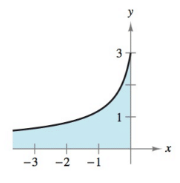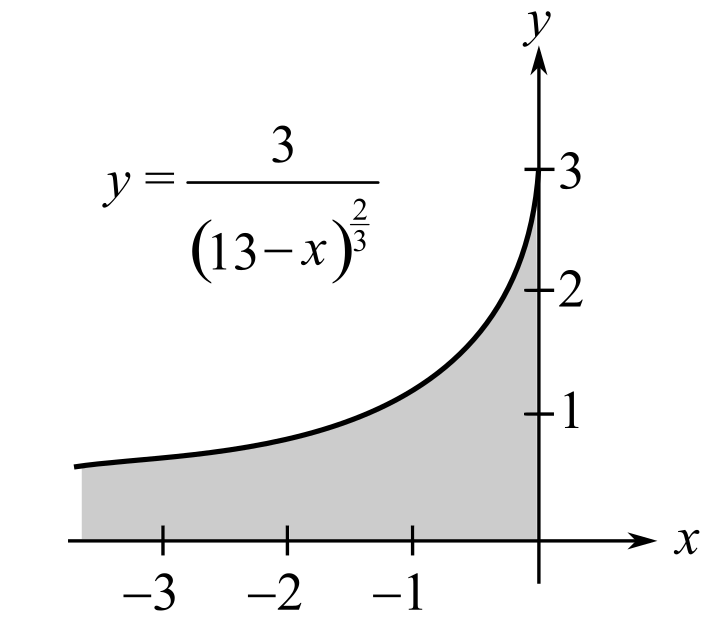Chapter 6, Problem 59RE### Calculus: An Applied Approach (Min...

10th Edition
Ron Larson
ISBN: 9781305860919

#### Solutions

Chapter
Section### Calculus: An Applied Approach (Min...

10th Edition
Ron Larson
ISBN: 9781305860919
Textbook Problem
1 views

# Area of a Region In Exercises 57–60, find the area of the unbounded shaded region. y = 3 ( 1 − 3 x ) 2 / 3To determine

To calculate: The area under the bounded region by the graph of the equation y=3(13x)23Explanation

Given Information:

The graph of the equation y=3(13x)23

Formula used:

From definition of improper integral.

If on the interval (,b], the function is continuous, then

bf(x)dx=limaabf(x)dx

The integral formula

eax=eaxa+C

Calculation:

Consider the provided expression:

03(13x)23dx

Use the definition of improper integral bf(x)dx=limaabf(x)dx

And simplify as:

03(13x)23dx=limbb03(13x)23dx

In the provided integral

Assume 13x=u

Now, differentiate

3dx=du<

### Still sussing out bartleby?

Check out a sample textbook solution.

See a sample solution

#### The Solution to Your Study Problems

Bartleby provides explanations to thousands of textbook problems written by our experts, many with advanced degrees!

Get Started

#### arcsin2x+arccos2x=1

Calculus: Early Transcendental Functions

#### Solve the equations in Exercises 126. x44x2=4

Finite Mathematics and Applied Calculus (MindTap Course List)

#### Inequalities Solve the inequality graphically. 143. 4x 3 x2

Precalculus: Mathematics for Calculus (Standalone Book)

#### In Exercises 49-62, find the indicated limit, if it exists. 57. limx2x2x6x2+x2

Applied Calculus for the Managerial, Life, and Social Sciences: A Brief Approach

#### Factor each expression in Problems 7-18 as a product of binomials. 11.

Mathematical Applications for the Management, Life, and Social Sciences

#### True or False: is a convergent series.

Study Guide for Stewart's Single Variable Calculus: Early Transcendentals, 8th

#### True or False: converges absolutely.

Study Guide for Stewart's Multivariable Calculus, 8th